# Proton 4: 2nd Degree, Polar Star (P4-21P)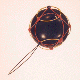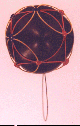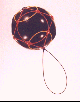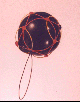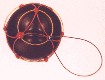The Proton Shell of 4 pins a single radical string for the second degree solution. The six structural loops form a cubic structure with one loop on each face of the cube. I have not modeled a stacked set of shells of the beryllium atom as yet. It should stack with the N5-41P shell to produce beryllium 9. N5-41P has an unusual quark distribution. In spite of the fact that I have found four different solutions for the P4 shell, the three solution sets below have similar symmetrical quark distributions. Only this solution is a bit lopsided in its quark distribution, and may represent the best fit with N5-41P in nature. Nature would tend to then favor stacking this shell with N5 over all the other P4 solutions. Beryllium 10, on the other hand, would have an N6 shell, which would clearly stack better with the charge-symmetry of the P4 shells given below.

## Stereograms of P4-21P ShellRequired Snap Points: 40 Available Snap Points: 39 Pinned Vector Bosons: 1 L1 = 20.23310855" (Structural) L2 = 16.52026974" (Filler) Model Diameter: 9.108101054" Loop Ratio (1:2): 1.224744442 Loop Equation: 6(L1) + 5(L2) = 204" Snap Point Equation: 6(4) + 5(3) = 39 A.S.P. Radical Strings:+ 1 P.V.B. = 40 R.S.P.

# Proton 4: 3rd Degree, Polar Star (P4-30P)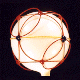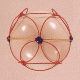A third degree solution set for P4. The first two photos give equatorial views, the third is a shot of the pole. This one also has a cubic core structure, but an equatorial string rounds things out.

## Stereograms of P4-30P Shell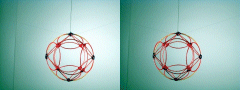Required Snap Points: 40 Available Snap Points: 40 Pinned Vector Bosons: 0 L1 = 19.10076345" (Structural) L2 = 27.01256519" (Equatorial) L3 = 15.59571352" (Filler) Model Diameter: 8.598366552" Loop Ratios: (1:2): 0.707106612 (1:3): 1.224744442 (2:3): 1.732050615 Loop Equation: 6(L1) + 1(L2) + 4(L3) = 204" Snap Point Equation: 6(4) + 1(4) + 4(3)= 40 R.S.P.

# Proton 4: 3rd Degree (P4-30)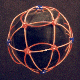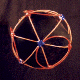The first model I built was lopsided, and I wasn't certain of the angles for mensuration, so I re-built it more carefully (still a bit warped). The pictures of the lopsided one are better (black balloon) though, so I included both. Had I discovered the polar star series earlier, I might not have so many alternate shell solutions to offer. This does appear to be a valid second degree solution, at least there are only two loop sizes required to construct it. The snap point equation is third degree, because the structural loops have either 4 or 6 snap points associated with them. The Up quarks on either side are closer together than on the preceding model, but otherwise the distribution of Up and Down quarks is quite similar. There should be some other differences in the charge currents attributable to the different structural geometry of this shell model.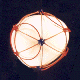## Stereograms of P4-30 ShellRequired Snap Points: 40 Available Snap Points: 40 Pinned Vector Bosons: 0 L1 = 27.90926031" (Structural) L2 = 27.90926031" (Structural) L3 = 16.11342461" (Filler) Model Diameter: 8.883793474" Loop Ratios: (1:2): 1.0 (1:3): 1.7320502 (2:3): 1.7320502 Loop Equation: (L1 = L2) 1(L1) + 4(L2) + 4(L3) = 204" Snap Point Equation: 1(4) + 4(6) + 4(3)= 40 R.S.P.

# Proton 4: 4th Degree (P4-42)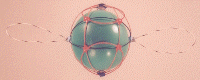This one has two radical strings and three loop sizes, and is similar to the solution given above. Three distinct loop sizes are used, but again, the snap points vary on the structural loops, making the snap point equation a fourth degree.

## Stereograms of P4-42 Shell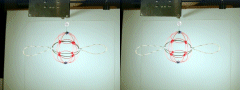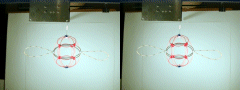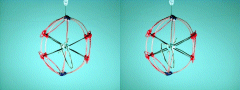Required Snap Points: 40 Available Snap Points: 38 Pinned Vector Bosons: 2 L1 = 29.72977409" (Structural) L2 = 29.72977409" (Structural) L3 = 17.16449909" (Filler) L4 = 21.02213137" (Filler) Model Diameter: 9.463281007" Loop Ratios: (1:2): 1.0 (1:3): 1.7320502 (1:4): 1.414213125 (2:3): 1.7320502 (2:4): 1.414213125 (3:4): 0.816496614 Loop Equation: (L1 = L2) 1(L1) + 4(L2) + 2(L3) + 1(L4) = 204" Snap Point Equation: 1(4) + 4(6) + 2(3) + 1(4)= 38 R.S.P. Radical Strings:+ 2 P.V.B. = 40 R.S.P.

Copyright 1997 by Arnold J. Barzydlo
Go Back